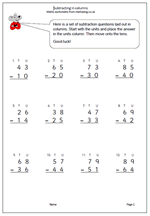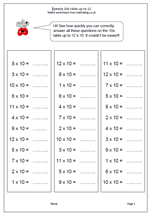## Subtraction in columnsIt is suggested in the new Maths Programme of Study that children in Year 2 should get used to recording addition nand subtraction in columns, both to support place value and to prepare for formal written methods with larger numbers in later years.

Here we have a subtraction worksheet laid out in columns. There is no ‘borrowing’ so it is a simple matter of subtracting the units and subtracting the tens.

Subtract 2-digit numbers in columns

## Learning the ten times table in Year 2Children are introduced to multiplication tables in Year 2 (6/7 yrs old) and should be fluent with the two times, five times and ten times tables by the end of the year. The ten times table is obviously the easiest to learn off by heart, but it can also be used to illustrate place value. The 5 in 50 has ten times the value of the 5 in 5.

Don’t ever suggest that all that needs to be done to multiply by ten is ‘add a nought’. This leads to real problems in later years when multiplying by decimals. Far better to say that the digit moves one place to the left and a zero is put in the units as a place holder.

Speedy 10x table